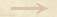Other Propositions Veloc-total-space-squared Other PropositionVeloc-total-space-squaredThe Velocity Area Theorem If Oresme's graphical representation of a 'uniformly difform quality' is applied to a velocity changing its intensity in proportion to the distance traversed, as it was assumed by Galileo in a period around 1604, it follows that the velocity of the entire motion is represented by the area of a triangle whose height is the distance traversed. This velocity thus grows in proportion to the square of the distance. Galileo expressed this conclusion as follows: If in the line of natural descent two unequal distances from the starting point of the motion are taken, the moments of velocity with which the body traverses these distances are to one another in double proportion of those distances. (Ms. Gal. 72, folio page 179v).Other Propositions Veloc-total-space-squared Other PropositionVeloc-total-space-squared